##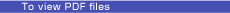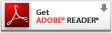Selected Papers: Research Activities in Laboratories of New NTT Fellows Part II

# Message Recovery Signature Schemes from Sigma-protocols

## Abstract

This paper presents a framework for constructing message recovery signature schemes from sigma-protocols. The heart of our construction is a redundancy function that adds some redundancy to a message that only a legitimately signed and recovered message can have. We characterize redundancy functions that make the resulting message recovery signature scheme proven secure. Our framework includes known schemes when the building blocks are given concrete implementations, so it presents insightful explanation into their structure.

† NTT Information Sharing Platform Laboratories Musashino-shi, 180-8585 Japan## 1. Introduction

Digital signatures have been a central research subject in cryptography and are widely used these days. Among the many theoretical issues to pursue, efficiency is of great concern in practice. It is typically measured by the size of the public key and the signatures and by the computational cost for signature generation and verification. This paper focuses on efficient digital signature schemes that yield short signatures.

A digital signature scheme yields either a digital signature with an appendix or with messae recovery. In the former case, a signed message looks like (σ, msg), where σ is the signature and msg is the message. Given (σ, msg), the verifier can determine whether σ is a correct signature that guarantees the authenticity and integrity of message msg. Naturally, the length of a signed message is the sum of the length of the message and the signature. In the latter case, a signed message is like (σ, n), where n is part of the message. The remaining part of the message is contained in signature σ, and anyone can recover the entire message. Because of this structure, one can expect a signed message to be shorter than a message in the case of a signature with appendix.

Typically, a message recovery signature proceeds as follows. The signer adds some redundancy to the message to sign it. This produces a signed message (σ, n). To verify the signed message, the verifier first recovers the entire message msg and then checks whether the recovered message has the correct redundancy. All that is required to recover the message is the public key, so anyone can do it. Furthermore, every random string (σ, n) will yield a string that looks like a redundant message (but is actually a random meaningless one). Accordingly, it is essential to choose redundancy that cannot be easily satisfied by the message recovered from forged (σ, n).

However, there are many message recovery signature schemes in the literature that do not specify or even characterize the necessary properties of the redundancy that makes the signature scheme proven secure. For RSA (Rivest, Shamir, and Adleman) signatures, there are some redundancy functions (also known as the padding method) such as PSS-R  that eventually achieves security against adaptive chosen message attacks  in the random oracle model . In –, Nyberg and Rouppel presented message recovery signature schemes whose security is based on the discrete-log problem, but no specific redundancy function was given, so its security is unclear. It was followed by some similar schemes, e.g., , also without specific redundancy functions. In , Abe and Okamoto presented a message recovery version of the Schnorr signature scheme  with a specific redundancy using random oracles but with a very complicated security analysis that results in a high cost for reduction to the discrete logarithm problem. In , Naccache and Stern constructed a message recovery signature scheme from DSA  with characterization of sufficient redundancy. Pintsov and Vanstone presented a message recovery version of the Schnorr signature scheme whose security is proven in the ideal cipher model, which is known as the ECPV scheme , . Many of these schemes are included in international standards such as .

The Fiat-Shamir transform  is a well-known methodology for obtaining a secure digital signature scheme. It converts a sigma-protocol ,  into a secure signature scheme in the random oracle model. A sigma-protocol is a two-party protocol where a prover interacts with a verifier to convince him that the prover knows a secret, which is often understood as a secret key corresponding to a public key, without leaking anything about the secret. More precisely, it is a public-coin three-round honest verifier zero-knowledge proof system that has special soundness and special honest verifier zero-knowledge. Such protocols are often used for interactive authentication. The Fiat-Shamir transform can be used to eliminate the interaction and enable the prover alone to create a transcript of the execution of the sigma-protocol so that it can act as evidence of his knowledge about the secret, like the interactive version. When an arbitrary message is bound to the transcript, one can see that only the person who knows the secret can create the transcript with the message. Thus, it works as a digital signature with appendix. However, it is not known whether such a technique is also useful for designing digital signature schemes with message recovery.

In this paper, we present a framework for constructing message recovery signature schemes from sigma-protocols. Our results show how to securely convolute part of a document into a message used in the sigma-protocol by using a redundancy function and eventually turn the sigma-protocol into a message recovery signature scheme that is adaptively chosen message secure in the random oracle model.

Importantly, we characterize the redundancy function that is sufficient to make the resulting message recovery signature scheme proven secure in the random oracle model. We then show two instantiations of the redundancy function that conforms to our requirements based on the random oracle model and the ideal cipher model. At 80-bit security with typical parameter settings, both instantiations shorten the signed message by 80 bits. Our framework yields known schemes, ECPV in particular, when the building blocks are given concrete implementations; hence, it presents insightful explanation into their structure.

## 2. Preliminaries

### 2.1 Notation

We consider uniform probabilistic algorithms (i.e., Turing machines) that take as input (the unary encoding of) a security parameter λ ∈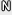and possibly other inputs and run in deterministic polynomial time in λ. We thus always implicitly require the size of the input to be bounded by some polynomial in λ. Adversarial behavior is modeled by non-uniform polynomial-time probabilistic algorithms, i.e., by algorithms that together with the security parameter λ also get a polynomial-sized auxiliary input auxλ. To simplify the notation, we usually let the dependency on λ (and on auxλ) remain implicit. By A(x), we denote the output distribution of a probabilistic algorithm A with input x and uniformly chosen randomness. By yA(x), we mean that algorithm A is executed on input x and the output is assigned to y. We may also denote it as yA(x; r) when the randomness r is to be explicitly noted. For any algorithm A, the output domain of A is denoted by Dom(A). Similarly, for any finite set S, we use the notation yS to denote that y is sampled uniformly from S, and yx means that the value x is assigned to y. By BitLen(S), we denote the number of bits used to present the largest element in S.

|X| is used in different ways. If X is a set, |X| denotes the cardinality of X. If X is a distribution or an algorithm, |X| denotes the number of elements in X with nonzero probability. If X is a variable whose actual value is taken from some range, |X| denotes the number of bits needed to present the maximum value in the range. (Hence, |X| does not necessarily equal [log2 X] for the actual value assigned to X.) Finally, if X is a string, |X| is the number of bits needed to present the string.

We use X := Y to define a new variable X with Y. For any two strings a and b, a||b denotes a string that concatenates a and b in order. By a||bc, we denote that string c is separated into two parts and assigned to a and b. The manner of separation depends on the context but may not be explicitly given when it is clear from the context.

P[y = A(x)] denotes the probability (taken over a uniformly distributed random tape) that A outputs y on input x, and we write P[x ← B : A(x) = y] for the (average) probability that A outputs y on input x when x is output by B: P[xB: A(x) = y] = Σx P[y = A(x)]P[x = B]. We also use natural self-explanatory extensions of this notation.

An oracle algorithm A is an algorithm in the above sense connected to an oracle in that it can write on its own tape an input for the oracle and tell the oracle to execute it. Then, in a single step, the oracle processes this input in the prescribed way and writes its output on the tape. We write AO when we consider A to be connected to the particular oracle O.

As is common practice, a value v(λ)∈R, which depends on the security parameter λ, is treated as negliible, denoted by v(λ) ≤ negl(λ) or vnegl, if ∀c > 0∃λ′∈∀λ ≥ λ′: v(λ) < 1/ λc.

### 2.2 Sigma-protocol

Let L⊂{0, 1}* be a language and RL be a binary relation associated with L. Let WL(x) for xL denote a set of witnesses for x, i.e., WL(x) = {|RL (x, w) = 1}. Let L be an instance generator for L that takes security parameter λ and chooses an instance xL and a witness wWL(x) with restriction |x| = λ. A sigma-protocol for language L is an honest verifier zero-knowledge proof system that consists of (probabilistic) polynomial-time algorithms (A, C, Z, V, E, M). These algorithms work as follows.

For (x, w) ← L(λ), the verifier is given x and the prover is given (x, w). The prover first sends the first message aA(x; t) to the verifier and the verifier challenges the prover by returning cC(x). The prover answers the challenge by sending zZ(w, t, c) to the verifier. The verifier accepts if a = V(x, c, z). (More generally, V can be an algorithm that takes (a, x, c, z) as input and returns 1 or 0 to report acceptance and rejection. Note, however, that it is essential for our purpose that a can be computed from (x, c, z) in polynomial time.) We assume perfect correctness, where the verifier accepts with probability 1 when both the prover and verifier are honest.

A sigma-protocol features special soundness. That is, there exists a deterministic polynomial-time algorithm E, called an extractor, that takes two acceptable transcripts with the same first message and different challenges, say (x, a, c, z) and (x, a, c′, z′) such that xL, cc′, V(x, c, z) = V(x, c′, z′) = a as input and outputs a witness w for x with probability 1 in polynomial time.

A sigma-protocol is honest verifier zero-knowlede. Namely, we assume that there exists a polynomial-time algorithm called a simulator, denoted by M, that takes xL and cC(x) as input and outputs (a, z) such that the distribution of simulated (a, c, z) is identical to the distribution of the real transcript generated by the honest prover and verifier.

A note on relaxation. We can allow a small error probability in the correctness but the resulting signature scheme inherits the error probability in its correctness. Similarly, E can be a probabilistic algorithm with negligible error probability. Also, the quality of zero-knowledge simulator M can be relaxed to statistical or computational rather than perfect. These relaxations affect the unforgeability of the resulting signature scheme.

### 2.3 Security model of message recovery signature scheme

To define the security precisely, we first must present a syntactical definition of message recovery signature schemes. The one shown below is a very standard definition, which can be seamlessly turned into the definition of ordinary signature schemes with appendix by letting the non-recoverable message n be the same as the entire message msg.

Definition 1. (Message recovery signature scheme) A message recovery signature scheme consists of three probabilistic polynomial-time algorithms (G, S, V).

G is a key generation algorithm that takes security parameter λ and outputs a public-key pk and a private-key sk. Associated with pk is a recoverable message space {0, 1}rec.

S is a signature-issuing algorithm that takes a private-key sk and a message msg and outputs a signature σ and the non-recoverable part of the message n.

V is a verification protocol that takes a public key pk and a signed message (σ, n) and outputs (accept, msg) or reject. It is required that (accept, msg) = V(pk, S(sk, msg)) for any pk and sk generated by G and for any msg.

A message recovery signature scheme is secure if it withstands an adversary that asks a legitimate signer to sign arbitrary messages and then attempts to forge a valid signed message never signed by the signer. Formal definition of such a chosen message adversary follows.

Definition 2. (Chosen message adversary) A (τsig, εsig, qs, qh) chosen message adversary Asig against the above message recovery signature scheme is an oracle Turing machine that launches a chosen message attack that consists of the following steps.

1. (pk, sk) ← G(λ)

2. (˜σ, ˜n ) ← AsigS,H(pk)

S is the signing oracle such that, for every signing query for a message, say msgi, it returns a legitimately generated signed message (σi, ni) by using private key sk. H is the random oracle that corresponds to hash function H in the signature scheme. Let T denote the signed messages (σi, ni) observed by S. The final output of Asig must satisfy (˜σ, ˜n )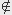T. Asig makes at most qs queries to S and at most qh queries to H and then stops within running time τsig. Then, V(pk, ˜σ,˜n ) outputs (accept, msg) for some msg with probability at least τsig. The probability is taken over all the random coins used in the chosen message attack.

## 3. Our construction

### 3.1 Redundancy function

The heart of our construction is the redundancy function, say Δ: Dom(A) × {0, 1}recD, that takes the first message a of the underlying sigma-protocol and a recoverable part of the message m and outputs a redundant message r in some specific domain D. More precisely, we assume a family of functions Δλ = {Δ} and its ensemble {Δλ}λ∈N that satisfy the following properties.

1. (Invertibility) For every Δ, there exists an inverse function Δ−1 that, given (a, r), outputs m. It is required that m = Δ−1(a, Δ(a, m)).

2. (Compactness) BitLen(Dom(A)) +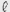recBitLen(D).

3. (Randomness) For every Δ and every m, the distribution of Δ(a, m) for uniformly chosen a is uniform.

4. (Collision resistance) Given Δ ← Δλ, any probabilistic algorithm running within time τ finds (a, m) and (a′, m′) such that Δ(a, m) = Δ(a′, m′) and (a, m) ≠ (a′, m′) only with probability, say εΔcoll(τ), which is negligible in λ if τ is polynomial in λ.

Invertibility is needed for our construction to work. Compactness is needed for the message recovery property to be meaningful. Without this property, the signed message of the resulting message recovery signature scheme would be longer than the one from the signature scheme with appendix. Randomness and collision resistance are needed for unforgeability of the resulting signature scheme.

One can relax the randomness property to be computationally indistinguishable from uniform. This will affect the unforgeability of the resulting signature scheme, as we mention later.

As we shall see in Section 4. εΔcoll(τ) may take some other parameters such as the maximum number of oracle queries, depending on its construction.

### 3.2 Our scheme

Given a sigma-protocol (A, C, Z, V, E, M) for L and a hash function H, we construct a message recovery signature scheme (G, S, V) as follows.

– (Key generation: G) Given λ, run (x, w) ← L(λ) and output x as a public key and w as a private key. Security parameter λ also determines the length of the recoverable partrec and the redundancy function Δ.

– (Signature generation: S) Given private key w and message msg, first chop msg as msg = m||n so that m {0, 1}rec. Then, compute aA(x; t), r ← Δ(a, m), cH(r, n), and zZ(w, t, c). Then, output (r, z, n) as a signed message.

– (Signature verification: V) Given x and (r, z, n), compute cH(r,n), aV(x, c, z), and m ← Δ−1(a, r). If r ← Δ(a, m), output (accept, m||,n). Otherwise, output reject.

Implementation note. Depending on the specific structure of function Δ, the signature verification can be more efficient than recomputing Δ. See also Subsections 4.1 and 4.2.

### 3.3 Proof of security

Theorem 1. (Security against chosen message attacks) If there exists (τsig, εsig, qs, qh)-adversary Asig for the message recovery signature scheme based on language L and an instance generator L, then there exists a (τw, εw) witness extraction adversary AL, where τw ≤ 8τ sim /,(εsig − 4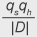)

and εw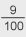.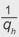(1 −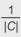) (1 − εcollΔ, sim).

Here, τsim is τsig + qsTsig, where Tsig is the sum of the running times of C, M, and Δ, and εcollΔ, sim is (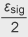) −1εΔcoll (2τsim).

Proof. This is proved by constructing AL from Asig using a forking lemma in a standard manner. Let xL be an instance generated by L(λ). Given x as input, AL works as follows.

1. Invoke Asig with x as a public key and a uniformly chosen random tape. If Asig terminates with a valid signed message (˜r,˜z, ˜n), proceed to the next step. Otherwise, repeat this step with the same x and a fresh random tape up to t1 times. Abort if never successful. Oracle queries are handled as follows.

– (Query msgi to S.) Execute ciC(x) and (ai, zi) ← M(x, ci). Then, chop msgi into mi||ni, compute ri ← Δ(ai, mi), and define H so that ci = H(ri, ni). If H has already been defined for such an input, abort. Otherwise, return (ri, zi, ni) as a signed message.

– (Query (rj, nj) to H .) If the input is fresh, select cj uniformly, define H as cj = H(ri, ni), and return cj. Otherwise, return the preliminarily defined value.

2. Let i* denote the index that H is asked (˜r, ˜n ). That is, ri* = ˜r, ni* = ˜n, and ci* = H(˜r, ˜n). Let ˜a denote ˜aV(x, ci*, ˜z). Move to the next step.

3. Invoke Asig with x and the random tape used in the successful run of the first step. The simulation for oracle S is unchanged. Oracle H is simulated identically up to the point that (˜r, ˜n )) is asked. From that moment, H is simulated with new randomness. If Asig terminates with a valid signed message (˜r, ˜z′, ˜n ), then proceed to the next step. Otherwise, repeat this step up to t2 times. Abort if never successful.

4. Let c′i* =H(˜r, ˜n), which was observed in the second step, and let ˜a′ be ˜a′← V(x, ci* , ˜z′). If ci* = ci* or ˜a˜a′, abort. Otherwise, compute wE(x, ˜a, ci*, ˜z, c′i* , ˜z′) and output w.

Now we estimate the running time and the probability of success of AL. First, the success probability is estimated as follows.

– First, we estimate the error probability of the oracle simulation. Observe that the simulation of S fails only if (ri, ni) has already been defined for H. Since the distribution of ni is arbitrarily determined by the adversary, we consider only the sufficient condition that ri has appeared so far.

Suppose that ri is uniformly chosen from D. Then, the probability that ri has appeared among the inputs to H is at most qh/|D|.

Next consider ri generated through Δ from uniformly chosen ai. Since we assume that the distribution of the output of Δ is uniform, the above probability does not change at all. (This is where the relaxation of randomness on Δ would have an effect. If the randomness is not perfect but just indistinguishable with some advantage, say εdistΔ(τ) for a polynomial-time distinguisher whose running time is , then the error probability of the simulation increases by εdistΔsim).)

Then, consider ai generated by M. As in the previous step, since the distribution of such ai is perfectly the same as uniform, the error probability does not change at all, either. (Again, this is where the relaxation on the zero-knowledge property of the underlying sigma-protocol matters. If the zero-knowledge simulation is only computational with some advantage, say at most εzk(τ) for a distinguisher whose running time is τ, then the error probability increases by εzksim).)

Therefore, the probability εserr that the simulation fails while handling qs queries is bound asThe simulation of random oracle H is obviously perfect unless the simulation of S fails.

– The success probability of Asig for fixed x is at least εsig/2 with probability 1/2 (over the choice of x) due to the heavy-row lemma of , . With the simulated signing oracle, the success probability reduces to εsig/2 − εserr. Accordingly, every run of Asig in the first step is successful with probability at least μ1: = (εsig/2 −εserr). By repeating the attempt up to t1: = 1/μ1 times, we get at least one valid (˜r, ˜z, ˜n ) at the end of the first step with probability greater than ε1 ≥1− (1 − μ1)1/μ1 ≥ 1 − e<−1 ≥ 3/5. Let Θ* denote the randomness used for simulating the random oracle for the i*-th and all subsequent queries in the successful run. Let Θ* denote all other randomness also used in the successful run.

– Over the choice of Θ*, the success probability of Asig for fixed Θ and x is at least εsig/4 with probability 1/2 (over the choice of Θ) due to the heavy-row lemma again. If we apply the same argument as in the first step, each attempt in the third step is successful with probability at least μ2: = εsig/4 − εserr. If we repeat this attempt up to t2: = 1/μ2 times, another valid signed message (˜r′, ˜z′, ˜n′;) is obtained with probability greater than ε2 ≥ 1 − (1 − μ2)1/μ2 ≥ 1 − e−1 ≥ 3/5. Furthermore, the outcome corresponds to the i*-th query to H, i.e., ˜r′ = ˜r and ˜n′ = ˜n, with probability at least 1/qh.

– In the fourth step, ci* = c′i* happens only with probability 1/|C|. Observe that, if ˜a˜a′, then we have ˜r = Δ(˜a, Δ−1(˜a, ˜r)) = Δ(˜a′, Δ−1(˜a′, ˜r)) which is a collision. Let εcollΔ,sim, denote the probability of such an event occurring. Since the running time of AL is less than τw= (t1 + t2) τsim, εcollΔ, sim, collsim can be upper bounded by εcollΔ, simw). It is, however, an overestimation. More precise estimation of the probability is at most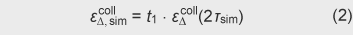because we have at most t1 trials for an independent choice of Δ, and the particular Δ chosen in the successful run in the first step is executed again in the third step of the simulation and the total running time with this particular Δ is 2τsim.

By summing up the above assessment, we get the total success probability of AL as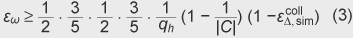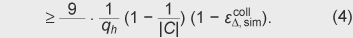Assuming that the time for generating randomness and table searching used for simulating H is free, the running time is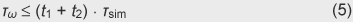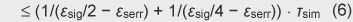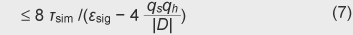## 4. Construction of Δ

### 4.1 In the random oracle model

We construct Δ and Δ−1 as follows.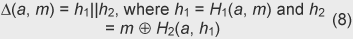H1: {0, 1}* → {0, 1}1 and H2: {0, 1}* → {0, 1}rec are hash functions modeled as independent random oracles.

To make the message recovery property meaningful,1 < |a| should hold. Note that, as we shall show in Lemma 2, settingrec too short would cause a security problem, i.e., it would result in a high probability of collision. Recommendable settings of1 andrec are1 =rec = |a|/2 to balance security and the benefit of message recovery. If one would like the length of the recoverable part to vary depending on the given message, the message must be polynomially long or some kind of padding must be applied so that the total length becomes polynomial in λ.

With the above instantiation, the verification procedure specified in Subsection 3.2 can be slightly more efficient. The modified signature verification procedure is as follows.

– (Signature verification: V) Given x and (r, z, n), compute cH(r, n), aV(x, c, z), and m ← Δ−1(a, r). Then, let h1||h2r. If h1 = H1(a, m), output (accept, m||n). Otherwise, output reject.

Namely, it replaces the testing r = Δ(a, m) with h1 = H1(a, m) and saves one computation of H2.

The above Δ satisfies the required properties listed in Subsection 3.1. Specifically, the following lemmas hold. The first three (invertibility, compactness, and randomness) are trivial and the last one (collision resistance) is followed by a detailed proof.

Lemma 1. (Invertibility, compactness, and randomness) The above Δ is invertible. In particular, the above Δ−1 is the inverse function. It is compact when1 is set so that BitLen(Dom(A)) ≥1. The output distribution of Δ is uniform over {0, 1}1+rec if H1 and H2 are independent random oracles.

Lemma 2. (Collision resistance) If1 andrec are polynomial in λ, the above Δ is collision resistant against polynomial-time adversaries that make a polynomial number of queries to H1 and H2. In particular, for any adversary asking q1 and q2 queries to H1 and H2, respectively, the probability of collision is upper bounded by ∈Δcoll(q1, q2) ≤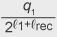++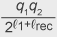+. +.

Proof. (of Lemma 2) We refine the notation for the input and output of H1 and H2 as follows. Let hi = H1(Ri, Mi) and cj = H2(Dj, ej) denote the i-th and j-th input/output of H1 and H2, respectively. We first define the notation and some terminology. Let L1 denote the set of queries and answers for H1, i.e., L1 = {(Ri, Mi, hi)|i = 1, ..., q1}. Define L2 = {(Dj, ej, cj)| j = 1, ..., q2} as well. Without loss of generality, we assume that every query is fresh, so every entry in L1 and L2 is unique. By (i, j), we denote a set that consists of the i-th entry of L1 and the j-th one of L2, i.e., (i, j) = (Ri, Mi, hi, Dj, ej, cj). We say that (i, j) is fully consistent if (Ri, hi) = (Dj, ej). Note that if (i, j) is fully consistent, it forms a correct computation of Δ. By FullCon (i, j) = true or false, we mean that (i, j) is or is not fully consistent, respectively. We also say that (i, j) is semi-consistent if Ri = Dj. A semi-consistent (i, j) may also be fully consistent but this is not necessary. By SemiCon(i, j) = true or false , we mean that (i, j) is or is not semi-consistent, respectively. Let τ(Hb, i) for b ∈1, 2 denote the time at which the i-th query is made to Hb. (It can be understood as the number of steps before the i-th query is made to oracle H1). Since no more than one query can be made at one step, τ(Hb, i) is strictly larger or smaller than τ(Hb′, i′) if ii′. We say that (i, j) is regular if τ(H1, i) < τ(H2, j); otherwise, it is irregular. The intuition behind this terminology is that anyone who computes Δ correctly should ask H1 first and then H2 in that order. Therefore, a normal computation of Δ yields regular and fully consistent (i, j). By Regular(i, j) = true or false , we mean that (i, j) is regular or irregular, respectively. We define function X(i, j) as X(i, j) = cjMi.

With this terminology, we can say that a collision Δ(Ri, Mi) = Δ(Ru, Mu) happens if and only if there exist j and υ such that

– (i, j) ≠ (u, υ),

– FullCon(i, j) = FullCon(u, υ) = true, and

hi||X(i, j) = hu||X(u, υ).

Accordingly, we say that (i, j) and (u, υ) collide if and only if the above conditions are satisfied. By Collision(i, j, u, υ) = true or false , we mean (i, j) and (u, υ) do or do not collide, respectively.

We classify entries in L2 by the value of ej. Namely, for every different value of ej in L2, we consider a set of indexes {υ|eυ = ej} and label such sets as Class1, Class2, .... In this way, every entry in L2 is associated with one class. Obviously, there are at most q2 classes. Let SameClass( j, υ) be true if and only if j and υ are in the same class, i.e., ej = eυ.

The above definitions lead simply to some facts.

Fact 1. If SameClass( j, υ) = false, then Collision(i, j, u, υ) = false.

If two consistent (i, j) and (u, υ) are in different classes, then hi = ejeυ = hu holds and they cannot collide. Moreover, if either of them is inconsistent, it cannot have a collision, either. Therefore, we consider the possibility of collision only within a class.

Fact 2. |{(i, j)|FullCon(i, j) = true}| ≤ q1.

Namely, there are at most q1 pairs of fully consistent queries. Suppose that (i, j) is fully consistent and (i, j′) is also fully consistent for some j′. Then, ej = hi = ej′ and Dj = Ri = Dj′ holds, and it means that the j-th and j′-th queries to H2 are identical, whereas we assumed that L2 contains no duplicate entries.

Fact 3. |{(i, j)|SemiCon(i, j) = true, ∧j∈Classk}| ≤ q1 for every Classk.

Namely, there are at most q1 semi-consistent pairs of queries with regard to each class of L2. Suppose that (i, j) and (i, j′) are both semi-consistent and j and j′ are in the same class. We then have Dj = Ri = Dj′ and ej = ej′. Hence, the j-th and j′-th entries in L2 are identical.

Suppose that (i, j) and (u, υ) are both semi-consistent and they are in the same class. These queries can collide. (On the other hand, if either of them is not semi-consistent or if they are in different classes, they cannot collide.) Regarding the regularity of (i, j) and (u, υ), one of the following cases must be true.

Case 1. Both are regular.

Case 2. One is regular and the other is irregular.

Case 3. Both are irregular.

A pair of regular queries, say (i, j), is either fully consistent (the case where (Ri, hi) is the input (Di, ei) to H2) or inconsistent (something else is given as input to H2). These cases are independent of the choice of the randomness of H1 and H2. If (i, j) is regular but inconsistent, there is no chance of it causing a collision with another pair of queries. Hence, we only need to consider fully consistent queries in the regular case. Below, we consider the probability of collision in each of the above cases.

Case 1. Consider regular and fully consistent queries (i, j) and (u, υ) in the same class. For a collision to occur, hi = hu and cjMi = cυ Mu are necessary. First of all, hi = hu happens with probability at most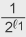because both hi and hu are uniformly chosen as a result of the true randomness of H1 and H2. Next, consider cjMi = cυMu. Since (i, j) and (u, υ) are regular, τ(Mi) < τ(cj) and τ(Mu) < τ(cυ) hold. Therefore, at least one of cj and cυ is independent of Mi and Mu. Since both cj and cυ are chosen uniformly, cjMi = cυMu holds with probability at most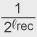. Thus, (i, j) and (u, υ) collide with probability at most. Let FullCon(Classk) denote the number of fully consistent queries in Classk. By summing up the probability for all the classes and applying Fact 2, we getCase 2. For every entry i in L1, there exists at most one entry, say j, in each class of L2 such that (i, j) is semi-consistent. (If there exists j′ in the same class such that (i, j′) is semi-consistent, then j = j′ because Ri = Dj = Dj′ and ej = ej′.) We consider the probability that the semi-consistent (i, j) in the class is irregular and collides with a regular and fully consistent (u, υ) in the same class. First of all, (i, j) must be fully consistent to have a collision. Therefore, it must satisfy hi = ej when hi is randomly and independently chosen, which in turn happens with probability at most. Second, cjMi = cυ Mu must hold. Since (i, j) is irregular, however, Mi can be set after the values of cj, cυ, and Mu have been seen, and this condition can be satisfied with probability 1 by setting Mi as Mi = cjcυMu. Note that there is only one regular and fully consistent (u, υ) in the class that causes cjMi = cυ Mu with (i, j). Otherwise, there would exist another regular and fully consistent (u′, υ′) in the same class and it would cause cjMi = cυ′Mu′. Then, (u, υ, u′, υ′) would have a collision. This has already been treated in Case 1.

One question is whether or not such a manipulated Mi can also be a candidate for a collision in other classes. We claim that if (i, j) in Classk has (u, υ) in Classk that satisfies cjMi = cυMu, then (i, j′) in Classk′ has (u′, υ′) in Classk′ that also causes cj′Mi = cυ′Mu′ only with probability .(Naturally, we suppose (i, j′) is irregular and semi-consistent and (u′, υ′) is regular and fully consistent so they fall into Case 2.) This happens only if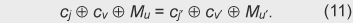Since (u, υ) and (u′, υ′) are regular, all cj cυMu cj′, and cυ′Mu′ are random and independent of each other. Hence, Eq.(11) is satisfied only with probability.

In summary, with regard to each query i in L1, we have a collision probability of at most+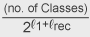+. Summing up the probabilities for all i in L1, we get the upper bound of the probability for Case 2 as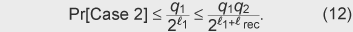Case 3. We consider the probability that, for an irregular semi-consistent query (i, j) in a class, there exists another irregular semi-consistent query (u, υ) in the same class that causes a collision.

First of all, (i, j) itself must be fully consistent. This is satisfied with probability at most. In addition, (u, υ) must be fully consistent and this happens with probability at most.

Next, we consider the number of (u, υ) in the class, say Classk, that collide with (i, j) with nonzero probability. We first claim that if j =υ , then (i, j) and (u, υ) cannot collide. Suppose that j =υ . Since both (i, j) and (u, υ) are semi-consistent, Ri = Dj = Dυ = Ru holds.

Since (i, j) and (u, υ) are distinct, (Ri, Mi) ≠ (Ru, Mu), so MiMu. If a collision happens, cjMi = cυMu must hold. But this is not possible because cj = and MiMu. We next claim that if (i, j) and (u, υ) satisfy cjMi = cυMu, then no other (u′,υ ) in the same class can cause cjMi = cυMu′. The reason is essentially the same as for the above claim. Since both (u, υ) and (u′, υ) are semi-consistent and in the same class, MuMu must be the case (otherwise, u and u′ are the same query in L1). Hence, cjMi = cυMu = cυMu cannot happen. From the above two claims, we can see that, for (i, j), and for every υ in Classk, there could exist at most one u that makes (i, j, u, υ) have a collision. Namely, there are at most |Classk| — 1 candidates of (u, υ) that have nonzero probability of collision with (i, j).

In summary, for every irregular semi-consistent (i, j), the probability that there exists an irregular semi-consistent (u, υ) that causes a collision is at most.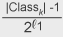. From Fact 3, we have at most q1 candidates of such (i, j) in a class. Thus, the probability of collision in each class is at most.. Summing up the probabilities for all classes and applying Σk |Classk| ≤ q2, we getSummary. From the bounds shown as in the Eqs. (10), (12), and (13), we get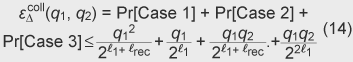as stated.

### 4.2 In the ideal cipher model

Let (SG, SE, SD) be a symmetric encryption such that: SG is a probabilistic algorithm that takes security parameter λ and outputs a secret key sk, SE is a (probabilistic) algorithm that encrypts input message msg and outputs a ciphertext ξ by using secret key sk, and SD is a decryption algorithm that takes ciphertext ξ and recovers plaintext msg by using secret key sk.

We model a symmetric encryption scheme as an ideal cipher in the following

SE and SD are oracles.

– Given a query (sk, msg), oracle SE does the following. If (sk, |msg|) is not recorded in Π, an initially empty list, select a new random permutation RP: {0, 1}|msg| → {0, 1}|msg| and return RP(msg). Then, record (sk, |msg|, RP) to Π. If (sk, |msg|) is in Π, simply return RP(msg) using the corresponding RP. Oracle SD does the reverse. That is, given a query (sk, ξ), if (sk, |ξ|) is not in Π, select a new random permutation RP: {0, 1}|ξ| → {0, 1}|ξ|, record (sk, |ξ|, RP) in Π, and return RP−1(ξ). If (sk, |ξ|) is in Π, simply return RP−1(ξ) using the corresponding RP.

We now construct Δ and Δ−1 as follows. Let str be a sufficiently long fixed string.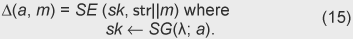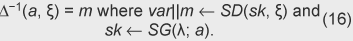Without loss of generality, we assume that SG takes randomness from the domain of a in the above construction. (Otherwise, a is preprocessed with an entropy smoothing method and then given to SG.) The output of SD is separated into two parts so that the length of the first part equals the length of the prefixed string str and the remaining part is taken as a recovered message.

In Δ−1, the recovered str is not used at all. It can be used to make the verification procedure slightly more efficient as follows.

– (Signature verification: V) Given x and (r, z, n), compute cH(r, n), aV(x, c, z), and m ← Δ−1(a, r). Then, let υar be the string obtained while computing Δ−1. If υar = str, output (accept, m||n). Otherwise, output reject.

Namely, it verifies whether or not the predetermined string str is recovered correctly.

We claim that the above construction conforms to our requirements. Again, the first three requirements are obviously satisfied. Collision resistance is stated with a proof below. Letstr be BitLen(str).

Lemma 3. (Invertibility, compactness, and randomness) The above Δ is invertible. In particular, the above Δ−1 is the inverse function. It is compact whenstr is set tostrBitLen(Dom(A)). The output distribution of Δ is uniform over {0, 1}str +rec.

Lemma 4. (Collision resistance) Ifstr andrec are polynomial in λ, the above Δ is collision resistant against polynomial-time adversaries that make a polynomial number of queries to SE and SD. In particular, for any adversary asking qd and qe queries to SE and SD, respectively, ∈Δcoll ≤ + holds.

Proof. Observe that a collision happens only if

– there exists at least one pair of the same value among the returned values from SE or

– there exists a message returned from SD whose leading part is str.

The probability of the former case is upper bounded by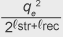as a result of the birthday paradox among at most qe randomly selected return values from SE. Since every value returned from SD is random, the probability that the second case occurs when at most qd queries are asked is at most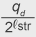. Summing up these bounds gives the upper bound as stated.

From these lemmas, recommendable settings would bestr =rec = BitLen(Dom(A))/2 to balance the efficiency of the message recovery property and unforgeability.

With this instantiation of the Δ function and the Schnorr identification scheme defined over a group over an elliptic-curve as the underlying sigma-protocol, the resulting message recovery signature scheme turns out to be the ECPV scheme presented in .

## 5. Conclusion and open problems

We presented a generic method that transforms a sigma-protocol into a message recovery signature scheme in the random oracle model with specific properties of the redundancy function. The framework allows one to build a new message recovery signature scheme in a modular fashion such that the sigma-protocol and the redundancy function are designed and analyzed in completely separate ways. Thus, one can now focus on designing the redundancy function with the shown properties and then automatically obtain a secure digital signature scheme with message recovery.

Two specific redundancy functions were shown and it was proved that one meets all the sufficient properties in the random oracle model while the other meets those in the ideal cipher model. Combined with a sigma-protocol in our framework, the first redundancy function yields a refined version of the ECAO message recovery signature scheme with a more understandable and convincing modular security proof. This can be regarded as an example that shows that our new framework can improve existing schemes by increasing their security. The second redundancy function yields an already known scheme, ECPV with another security proof. Thus, our framework gives another insightful explanation into an existing scheme and validates its design. These examples show the usefulness of our framework.

One of the remaining challenges is to construct the redundancy function without using idealized assumptions. Another challenge is to find another framework that yields shorter signed messages. One essential question is whether the redundancy is unavoidable or not for any message recovery signature schemes. Another direction of research includes relaxing the requirements for the redundancy function or even finding necessary and sufficient conditions.

## References

 M. Bellare and P. Rogaway, “The exact security of digital signatures—how to sign with RSA and Rabin,” in U. Maurer, editor, Advances in Cryptology, EUROCRYPT '96, Vol. 1070 of Lecture Notes in Computer Science, pp. 399–416, Springer-Verlag, 1996. S. Goldwasser, S. Micali, and R. Rivest, “A digital signature scheme secure against adaptive chosen-message attacks,” SIAM Journal on Computing, Vol. 17, No. 2, pp. 281–308, Apr. 1988. M. Bellare and P. Rogaway, “Random oracles are practical: a paradigm for designing efficient protocols,” First ACM Conference on Computer and Communication Security, pp. 62–73, Association for Computing Machinery, 1993. K. Nyberg and R. A. Rueppel, “A new signature scheme based on the DSA giving message recovery,” in Proceedings of the First ACM Conference on Computer and Communications Security, 1993. K. Nyberg and R. A. Rueppel, “Message recovery for signature schemes based on the discrete logarithm problem,” in Alfredo De Santis, editor, Advances in Cryptology, EUROCRYPT '94, Vol. 950 of Lecture Notes in Computer Science, pp. 182–193, Springer-Verlag, 1995. K. Nyberg and R. A. Rueppel, “Message recovery for signature schemes based on the discrete logarithm problem,” Designs, Codes and Cryptography, Vol. 7, No. 1–2, pp. 61–81, 1996. A. Miyaji, “A message recovery signature scheme equivalent to DSA over elliptic curves,” in Advances in Cryptology, Asiacrypt '96, Vol. 1163 of Lecture Notes in Computer Science, pp. 1–14, Springer-Verlag, 1996. M. Abe and T. Okamoto, “A signature scheme with message recovery as secure as discrete logarithm,” IEICE Transaction of Fundamentals of Electronic Communications and Computer Science, E84-A(1), Jan. 2001 (presented at ASIACRYPT '99). C. P. Schnorr, “Efficient signature generation for smart cards,” Journal of Cryptology, Vol. 4, No. 3, pp. 239–252, 1991. D. Naccache and J. Stern, “Signing on a postcard,” Financial Cryptography 2000, Vol. 1962 of Lecture Notes in Computer Science, pp. 121–135, Springer-Verlag, 2001. U.S. Department of Commerce, National Institute of Standards and Technology, “Digital signature standard,” FIPS PUB 186-2, 2000. L. Pintsov and S. Vanstone, “Postal revenue collection in the digital age,” in Financial Cryptography 2000, Vol. 1962 of Lecture Notes in Computer Science, pp. 105–120, Springer-Verlag, 2000. D. Brown and D. Johnson, “Formal security proofs for a signature scheme with partial message recovery,” in CT-RSA 2001, Vol. 2020 of Lecture Notes in Computer Science, pp. 126–142, Springer-Verlag, 2001. ISO/IEC 9796-3: Information technology—Security techniques—Digital signature schemes giving message recovery—Part 3: Discrete logarithm based mechanisms, 2nd Edition, JTC 1/SC 27, 2006. A. Fiat and A. Shamir, “How to prove yourself: Practical solutions to identification and signature problems,” in A. M. Odlyzko, editor, Advances in Cryptology, CRYPTO '86, Vol. 263 of Lecture Notes in Computer Science, pp. 186–199, Springer-Verlag, 1987. R. Cramer, “Modular Design of Secure yet Practical Cryptographic Protocols,” Ph.D. thesis, Aula der Universiteit, 1996. J. Garay, P. MacKenzie, and K. Yang, “Strengthening zero-knowledge protocols using signatures,” in E. Biham, editor, Advances in Cryptology, Eurocrypt '03, Vol. 2656 of Lecture Notes in Computer Science, pp. 177–194, Springer-Verlag, 2003. Full version available from IACR e-print archive 2003/037. D. Pointcheval and J. Stern, “Security arguments for digital signatures and blind signatures,” Journal of Cryptology, Vol. 13, No. 3, pp. 339–360, 2000.Masayuki Abe Distinguished Research Scientist, Okamoto Research Laboratory, NTT Information Sharing Platform Laboratories. He received the B.E. and M.E. degrees in electrical engineering from the Science University of Tokyo, Tokyo, in 1990 and 1992, respectively, and Ph.D. degree from the University of Tokyo, Tokyo, in 2002. He joined NTT Network Information Systems Laboratories in 1992 and engaged in the development of fast algorithms for cryptographic functions and their software/hardware implementation and the development of a software cryptographic library. He is a member of the Institute of Electronics, Information and Communication Engineers (IEICE) of Japan and the Information Processing Society of Japan. Tatsuaki Okamoto Research Fellow, Okamoto Research Laboratory, NTT Information Sharing Platform Laboratories. He received the B.E., M.E., and Dr.Eng. degrees from the University of Tokyo, Tokyo, in 1976, 1978, and 1988, respectively. He is a member of IEICE of Japan and the International Association for Cryptologic Research. Koutarou Suzuki Research Scientist, Information Security Project, NTT Information Sharing Platform Laboratories. He received the B.S., M.S., and Ph.D. degrees from the University of Tokyo, Tokyo, in 1994, 1996, and 1999, respectively. He joined NTT Information Sharing Platform Laboratories in 1999. He has been engaged in research on public key cryptography, especially on cryptographic protocols and digital signatures. He is a member of IEICE and the Information Processing Society of Japan. He received the SCIS Paper Award from IEICE in 2002.

↑ TOP